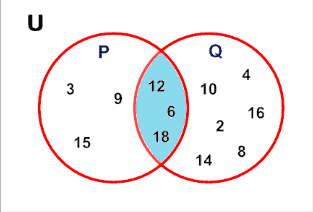# Python Set intersection: The Complete Guide

Before going through the intersection() method, we should know what an intersection is. It refers to the common elements of two given sets. This means if there are two sets and they have some common elements, then they are known as the intersection of both elements.## Python Set intersection()

Python set intersection() is a built-in method used to find the intersection between given sets. The set intersection() method finds the intersection of two or more two sets using this method. The intersection() method returns the set that contains the similarity between two or more sets.

The set intersection() method returns the set that contains the similarity between two or more sets. That means that the returned set contains only items in both sets or all sets if the comparison is made with more than two sets.

So, the intersection() method is used to find the intersection between given sets. We can see the intersection of two or more two sets using this method.

### Syntax

```set1.intersection(set2,set3,set4...)
```

Here, set1 is one set we want to find intersections, and set2,set3,set4, and so on are other sets.

### Return Value

The intersection() method returns the intersection of set1 with all other sets. If no argument is passed as a parameter to the intersection() function, it returns a shallow copy of the set1.

### Example

See the following code example.

```# app.py

# Declaring two sets

# Even nums between 2 and 10
set1 = {2, 4, 6, 8, 10}
# Multiple of 3 between 1 to 10
set2 = {3, 6, 9}

# priting both the sets
print("Set1 is: ", set1)
print("Set2 is : ", set2)
# Now we will find intersection of these two sets
print("intersection of set1 and set2 is: ", set1.intersection(set2))
```

#### Output

```Set1 is:  {2, 4, 6, 8, 10}
Set2 is :  {9, 3, 6}
intersection of set1 and set2 is:  {6}```

So, here in this example, we can see that we have declared two sets of even numbers and multiples of 3. Now we have found an intersection of them. As we can see intersection should be 6 because only 6 is common in both the sets; the output is also 6.

## The intersection of more than two elements

See the following code.

```# app.py

# Declaring two sets
# Even nums between 2 and 10
set1 = {2, 4, 6, 8, 10}
# Multiple of 3 between 1 to 10
set2 = {3, 6, 9}
# prime numbers between 1 to 10
set3 = {2, 3, 5, 7}
# odd numbers between 1 to 10
set4 = {1, 3, 5, 7, 9}

# Now we will find intersection of some sets

# intersection of set1 and set2
print("intersection of set1 and set2 is: ", set1.intersection(set2))
# intersection of set3 and set4
print("intersection of set3 and set4 is: ", set3.intersection(set4))
# intersection of set2 and set3
print("intersection of set2 and set3 is: ", set2.intersection(set3))
# intersection of set2 and set3,set4
print("intersection of set2 and set3,set4 is: ", set2.intersection(set3, set4))
# intersection of set1 and set2,set3,set4
print("intersection of set1 and set2,set3,set4 is: ",
set1.intersection(set2, set3, set4))
```

#### Output

```intersection of set1 and set2 is:  {6}
intersection of set3 and set4 is:  {3, 5, 7}
intersection of set2 and set3 is:  {3}
intersection of set2 and set3,set4 is:  {3}
intersection of set1 and set2,set3,set4 is:  set()
```

In this example, we can see that we have found the intersection of set1 and set2, set3 and set4, set2, and set3, so according to that, all common elements are printed. Similarly, the intersection of set2 and set3, set4, are 3 that are printed.

Also, when we find the intersection of set2, set3, and set4 with set1, the result is None because there is no common element in them.